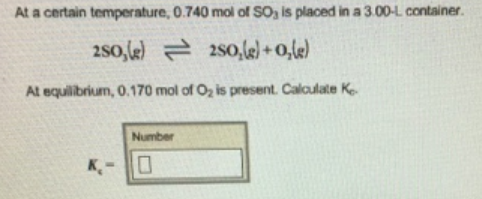# Problem: At a certain temperature, 0.740 mol of SO3 is placed in a 3.00-L container. 2SO3(g)  ⇌ 2SO2(g) + O2(g)At equilibrium, 0.170 mol of O2 is present. Calculate Kc.

###### FREE Expert Solution
87% (146 ratings)###### Problem Details

At a certain temperature, 0.740 mol of SO3 is placed in a 3.00-L container.

2SO3(g)  ⇌ 2SO2(g) + O2(g)

At equilibrium, 0.170 mol of O2 is present. Calculate Kc.